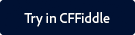Note:

You can find the CFFiddle demo of this function and other spreadsheet functions as part of a project that is shared with you.

Click the button below to launch CFFiddle.To copy the project in your workspace in CFFiddle, follow the steps below:

2. Navigate to the project in the left pane.
3. Once you make some changes in any cfm in the project, a pop up displays asking you to save the project.
4. Give the project a suitable name and click Save.

#### Description

Gets the formula for an Excel spreadsheet object cell, or all formulas for the object.

#### Returns

If the parameters include the row and column: a string containing the formula. If the function has the spreadsheetObj parameter, an array containing structures for each formula.

#### Category

Microsoft Office Integration

#### History

ColdFusion 9: Added the function.

#### Parameters

Parameter

Description

The Excel spreadsheet object from which to get the formula.

row

The row number of the cell from which to get the formula.

column

The column number of the cell from which to get the formula.

#### Usage

If you specify only the spreadsheetObj parameter, the function returns an array of the structures with the following contents. The array has one entry for each cell that contains a formula.

Field

Valid values

formula

The formula for the cell.

row

The row number of the cell.

column

The column number of the cell.

#### Example

The following example sets a cell formula, and gets the cell formula and value.

 //Create a new Excel spreadsheet object. theSheet=SpreadsheetNew(); //Set the values of column 3 rows 1-10 to the row number. for (i=1; i<= 10; i=i+1) SpreadsheetSetCellValue(theSheet,i,i,3); //Set the formula for the cell in row 11 column 3 to be the sum of //Columns 1-10. SpreadsheetSetCellFormula(theSheet,"SUM(C1:C10)",11,3); //Get the formula from the Excel spreadsheet object. theValue=SpreadsheetGetCellFormula(theSheet,11,3); //Get the value of row 11 column 5 from the Excel spreadsheet object. theValue=SpreadsheetGetCellValue(theSheet,11,3); Row 11, Column 3 value: #SpreadsheetGetCellValue(theSheet,11,3)#
Row 11, Column 3 formula: #SpreadsheetGetCellFormula(theSheet,11,3)#Twitter™ and Facebook posts are not covered under the terms of Creative Commons.